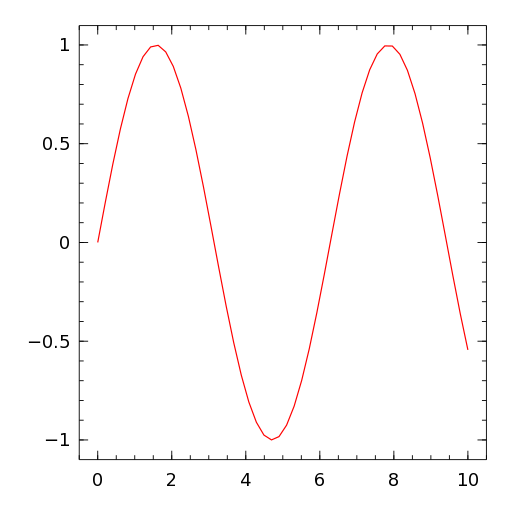# PlotInset¶

PlotInset(p::Tuple, q::Tuple, plot)

This PlotComponent can be used to embed plot in a different plot. p and q must be tuples of length 2 giving the position of the upper and lower corner in the parent plot. The coordinates in p and q are normalized to range between 0 and 1. The parent plot is given by the third argument plot and must be of type PlotContainer.

## Example¶

x = linspace(0, 10)
p1 = plot(x, x.^2)
p2 = plot(x, sin.(x), color="red")
inset = PlotInset((0.1, 0.6), (0.7, 0.9), p2)